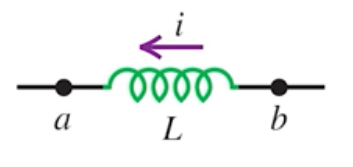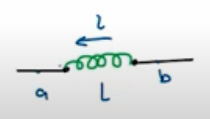Need Help?

Subscribe to Physics 2

###### \${selected_topic_name}
• Notes
• Comments & Questions

$\begin{array}{l}{\text { A toroidal solenoid has } 500 \text { turns, cross-sectional area }} \\ {6.25 \mathrm{cm}^{2}, \text { and mean radius } 4.00 \mathrm{cm} . \text { (a) Calculate the coil's self- }} \\ {\text { inductance. (b) If the current decreases uniformly from } 5.00 \mathrm{A} \text { to }}\end{array}$

$\begin{array}{l}{2.00 \mathrm{A} \text { in } 3.00 \mathrm{ms} \text { , calculate the self-induced emf in the coil. (c) The }} \\ {\text { current is directed from terminal } a \text { of the coil to terminal } b . \text { Is the }} \\ {\text { direction of the induced emf from } a \text { to } b \text { or from } b \text { to } a ?}\end{array}$

(a) $L=\frac{M_{0} N^{2} A}{2 \pi v}$

$=\frac{4 \pi*10^{-7} * 500^{2} * 6.25 * 10^{-4}}{2 \pi(0.04)}=7.81 * 10^{-4} \mathrm{H}$

(b) $\varepsilon=L \frac{d_ i}{d_ t}=7.81*10^{-4} *\left(\frac{5-2}{3 \times 10^{-3}}\right)=0.781 \mathrm{V}$

$\begin{array}{l}{\text { When the current in a toroidal solenoid is changing at a }} \\ {\text { rate of } 0.0260 \mathrm{A} / \mathrm{s}, \text { the magnitude of the induced emf is } 12.6 \mathrm{mV} \text { . }} \\ {\text { When the current equals } 1.40 \mathrm{A} \text { , the average flux through each turn }} \\ {\text { of the solenoid is } 0.00285 \mathrm{Wb} \text { . How many turns does the solenoid }} \\ {\text { have? }}\end{array}$

$L=\frac{N \Phi_{B}}{i}$

$L=\frac{\varepsilon}{d i/{d t}}$

$\rightarrow \quad N=\frac{\varepsilon_{i}}{\Phi_{B} | \frac{d _i}{d _t}|}$$=\frac{12.6 * 10^{-3} * 1.4}{0.00285*0.026}$

$=238 \text { trms. }$

$\begin{array}{l}{\text { The inductor in Fig. } \mathrm{E} 30.11 \text { has }} \\ {\text { inductance } 0.260 \mathrm{H} \text { and carries a current in }} \\ {\text { the direction shown that is decreasing }} \\ {\text { at a uniform rate, } d i / d t=-0.0180 \mathrm{A} / \mathrm{s}}\end{array}$

$\begin{array}{l}{\text { (a) Find the self-induced emf. (b) Which }} \\ {\text { end of the inductor, } a \text { or } b, \text { is at a higher potential? }}\end{array}$$|\varepsilon|=L\left|\frac{d i}{d t}\right|$

(a) $|\varepsilon|=0.26 *(0.018)=4.68 * 10^{-3} \mathrm{V}$

(b) $a \rightarrow$$\text { higher potential }$No comments yet